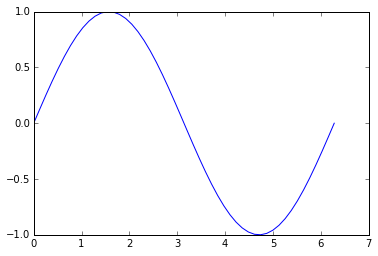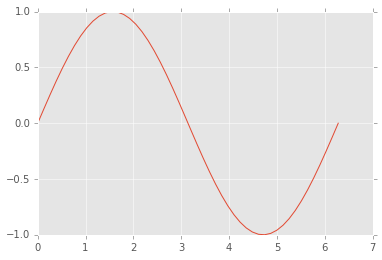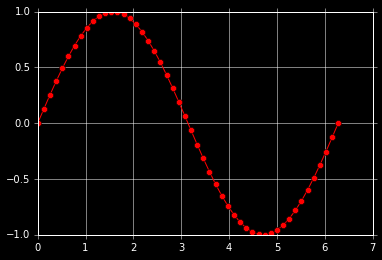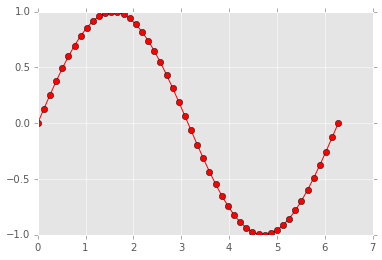# 使用 style 来配置 pyplot 风格¶

In :
import matplotlib.pyplot as plt
import numpy as np

%matplotlib inline


stylepyplot 的一个子模块，方便进行风格转换， pyplot 有很多的预设风格，可以使用 plt.style.available 来查看：

In :
plt.style.available

Out:
[u'dark_background', u'bmh', u'grayscale', u'ggplot', u'fivethirtyeight']
In :
x = np.linspace(0, 2 * np.pi)
y = np.sin(x)

plt.plot(x, y)

plt.show()In :
plt.style.use('ggplot')

plt.plot(x, y)

plt.show()In :
with plt.style.context(('dark_background')):
plt.plot(x, y, 'r-o')
plt.show()In :
with plt.style.context(('dark_background')):
pass
plt.plot(x, y, 'r-o')
plt.show()In :
plt.style.use(['dark_background', 'ggplot'])

plt.plot(x, y, 'r-o')
plt.show()mpl_configdir 的位置可以这样查看：

In :
import matplotlib
matplotlib.get_configdir()

Out:
u'c:/Users/Jin\\.matplotlib'

axes.titlesize : 24
axes.labelsize : 20
lines.linewidth : 3
lines.markersize : 10
xtick.labelsize : 16
ytick.labelsize : 16

plt.style.use('presentation')